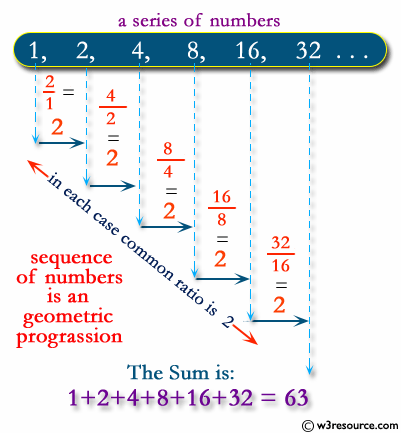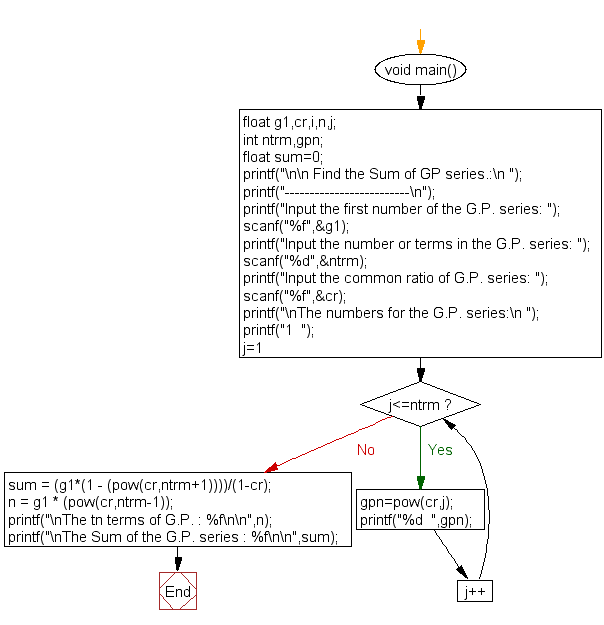﻿ C Program: Find the Sum of GP series - w3resource# C Exercises: Find the Sum of GP series

## C For Loop: Exercise-52 with Solution

Write a program in c to find the Sum of GP series.

Pictorial Presentation:Sample Solution:

C Code:

``````#include <stdio.h>
#include <math.h>

void main()
{

float g1,cr,i,n,j;
float ntrm,gpn;
float sum=0;

printf("\n\n Find the Sum of GP series.:\n ");
printf("-------------------------\n");

printf("Input the first number of the G.P. series: ");
scanf("%f",&g1);

printf("Input the number or terms in the G.P. series: ");
scanf("%f",&ntrm);

printf("Input the common ratio of G.P. series: ");
scanf("%f",&cr);

/*-------- generate G.P. series ---------------*/
printf("\nThe numbers for the G.P. series:\n ");
printf("%f ",g1);
sum=g1;

for(j=1;j<ntrm;j++)
{
gpn=g1*pow(cr,j);
sum=sum+gpn;
printf("%f  ",gpn);
}
/*-------- End of G.P. series generate ---------------*/
printf("\nThe Sum of the G.P. series : %f\n\n",sum);
}
```
```

Sample Output:

```Input the first number of the G.P. series: 3
Input the number or terms in the G.P. series: 5
Input the common ratio of G.P. series: 2

The numbers for the G.P. series:
3.000000 6.000000  12.000000  24.000000  48.000000
The Sum of the G.P. series : 93.000000
```

Flowchart:C Programming Code Editor:

Improve this sample solution and post your code through Disqus.

What is the difficulty level of this exercise?

Test your Programming skills with w3resource's quiz.

﻿

## C Programming: Tips of the Day

You are seeing the ffffff because char is signed on your system. In C, vararg functions such as printf will promote all integers smaller than int to int. Since char is an integer (8-bit signed integer in your case), your chars are being promoted to int via sign-extension.

Since c0 and 80 have a leading 1-bit (and are negative as an 8-bit integer), they are being sign-extended while the others in your sample don't.

```char    int
c0 -> ffffffc0
80 -> ffffff80
61 -> 00000061
```
Here's a solution:
```char ch = 0xC0;
printf("%x", ch & 0xff);
```

This will mask out the upper bits and keep only the lower 8 bits that you want.

Ref : https://bit.ly/3vOLizM